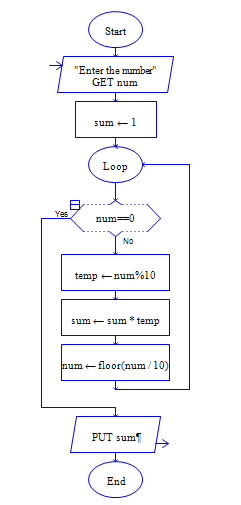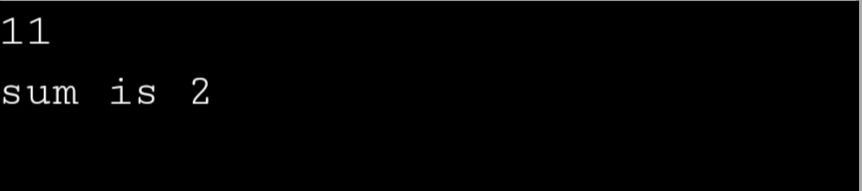# Product of Digits of the number using Python

Get a number and multiply the digits of the number.

Sample Input 1:

Enter the number: 234

Sample Output 1:

24

#### Flow Chart Design#### Program or Solution

``` num = int(input("enter a number"))n = numproduct = 1while n != 0:    rem = n % 10    product = product * rem    n = n // 10print(product) ```

#### Output#### Program Explanation

initialize product is equal to 1

calculate the remainder of a number by doing number % 10,  multiply the remainder with total and divide the number by the 10 and repeat the above steps till number becomes zero.

Print the product, you will get the product of digits of the number.